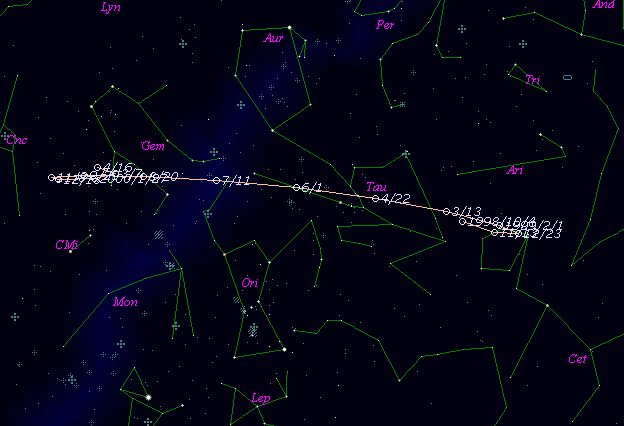\$B%j%K%"WB@1(B

P/1998 VS24 ( LINEAR )\$B%W%m%U%#!<%k(B

 \$BH/8+F|(B 1998\$BG/(B11\$B7n(B10\$BF|(B \$BH/8+8wEY(B 18.5\$BEy(B \$BH/8+ Lincoln Laboratory Near-Earth Asteroid Research project\$B50F;MWAG(B

The following improved orbital elements by Kenji Muraoka, are
from 41 observations 1998 Oct. 24 to 1999 Jan. 14, perturbations
by 9 Planets, Moon and 5 minor planets were taken into account.
The mean residual is +/- 0.67 arc seconds.

Epoch  =  1998 Nov.  3.0  TT       JDT = 2451120.5
T  =  1998 Nov.  2.74288       +/- 0.42378 (m.e.) TT
Peri. =  244.49809                +/- 0.37616
Node  =  159.20540                +/- 0.33259   (2000.0)
Incl. =    5.03127                +/- 0.01276
q  =    3.4053810              +/- 0.0003107 AU
e  =    0.2439534              +/- 0.0000458
a  =    4.5041946              +/- 0.0003072 AU
n  =    0.10310467             +/- 0.00001055
P  =    9.559                  +/- 0.0009781  years
(+/- 0.36 day)\$B@1?^(B\$B8wEYJQ2=(B

m1 = 11.0 + 5 log\$B&\$(B + 10.0 log r\$B50F;MWAG\$OB<2,7r<#;a\$N7W;;\$K\$h\$k\$b\$N\$G\$9!#(B \$B@1?^\$O(B StellaNavigator Ver.2.0 for Windows (\$B%"%9%H%m%"!<%D(B \$BJTCx(B / \$B%"%9%-!<=PHG6I4)(B) \$B\$G:n@.\$7\$?\$b\$N\$G\$9!#(B \$B8wEY%0%i%U\$O(BComet for Windows\$B\$G:n@.\$7\$?\$b\$N\$G\$9!#(B